# Adding + natural numbers - math problems

#### Number of problems found: 53

• The sumThe sum of the squares of two immediately following natural numbers is 1201. Find these numbers.
• Two numbers 7The sum of two consecutive even numbers is 30. Find the numbers.
• Sum of the seventeen numbersThe sum of the 17 different natural numbers is 154. Determine the sum of the two largest ones.
• Find the sumFind the sum of all natural numbers from 1 and 100, which are divisible by 2 or 5
• Three numbers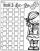How much we increases the sum of three numbers when the first enlarge by 14, second by 15 and third by 16? Choose any three two-digit numbers and prove results.
• Hello adding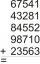Fill letters instead of digits so the indicated sum (equal letters represent equal digits). What number is hidden under the letter J? A A H A H O A H O J -------------------------- 4 3 2 1
• Parenthesis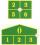Fill in the examples correctly brackets: A) 7.9+12:3-2 =23 B) 7.9+12:3-2=75
• House numbering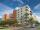The residential house has three entrances numbered even numbers, successive immediately behind. The sum of the two numbers on the outside entrances is 68. Calculate the middle of these three numbers.
• AlgebrogramSolve algebrogram for sum of three numbers: BEK KEMR SOMR ________ HERCI
• Finite arithmetic sequenceHow many numbers should be inserted between the numbers 1 and 25 so that all numbers create a finite arithmetic sequence and that the sum of all members of this group is 117?
• Sum of odd numbersFind the sum of all odd integers from 13 to 781.
• Identity123456789 = 100 Use only three plus or minus characters to correct previous identity/equation.
• Summands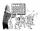Find two summands of the number 42, so that its product is minimized.
• SummandOne of the summands is 145. The second is 10 more. Determine the sum of the summands.
• Self-counting machineThe self-counting machine works exactly like a calculator. The innkeeper wanted to add several three-digit natural numbers on his own. On the first attempt, he got the result in 2224. To check, he added these numbers again and he got 2198. Therefore, he a
• FractionFraction ? write as fraction a/b, a, b is integers numerator/denominator.
• AddingDivide number 135 into two additions so that one adds 30 more than 2/5 of the other add. Write the bigger one.
• On the floor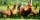You enter the room and there are 2 dogs, 4 horses, 1 giraffe and one duck on the bed, 3 chickens are flying over the chair. How many feet are on the floor?
• Pages countingThere are pages numbered from 2 to 104 in the book. How many digits have to be printed to number the pages?
• MistakeNicol mistake when calculate in school. Instead of add number 20 subtract it. What is the difference between the result and the right result?

Do you have an interesting mathematical word problem that you can't solve it? Submit a math problem, and we can try to solve it.

We will send a solution to your e-mail address. Solved examples are also published here. Please enter the e-mail correctly and check whether you don't have a full mailbox.

Please do not submit problems from current active competitions such as Mathematical Olympiad, correspondence seminars etc...

Adding Problems. Natural numbers - math problems.